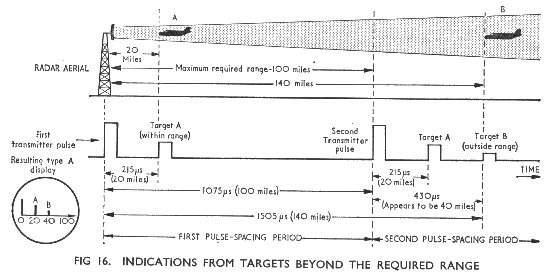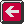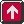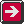Main Radar Home Radar theory Home AP3302 Pt3 Contents AP3302 Pt3 Section 1 Contents AP 3302 Pt. 3 Section 1 CHAPTER 3 Factors affecting the performance of Pulse-Modulated Radars of the c.r.t. screen is normally sufficient to retain the indication of the target B all the time and, to the operator, target B appears to be at a range of 40 miles (Fig 16).To avoid this, the pulse spacing is made very much longer than its minimum value of 1075 us, i.e. the p.r.f. is reduced well below 930 p.p.s. The c.r.t. timebase trace however is still adjusted for a sweep time of 1075 us. If a p.r.f. of 200 p.p.s. is selected the result will be as shown in Fig. 17. Any target beyond 100 miles range will return an echo after 1075 us, when the timebase trace has been switched off, and so will produce no indication on the c.r.t. screen. The same reasoning applies for any required radar range, but for smaller ranges the time intervals are less and the p.r.f. may be increased. Thus the longer the required range the lower must be the p.r.f. b. Scanning speed. If the aerial scanning speed is high and the p.r.f. is low some targets may be missed because, in the time interval between pulses, the aerial will have turned through a certain angle. Thus the higher the scanning speed the higher must be the p.r.f. Usually however the p.r.f. is decided by other factors and it is then the scanning speed which is adjusted to suit the selected p.r.f. The speed of scan is related to the p.r. f. by the expression Frq (Theta), where Fr is the p.r.f. in p.p.s. and q (Theta) is the half-power beam width in degrees. For a beam width of 1o and a p.r.f. of 500 p.p.s. the scanning speed must be less than 500 x 1o = 500o per second or l.4 r.p.s. (84 r.p.m.). This ensures that at least one pulse per beam width is transmitted. c. Mean power available. Given a certain available mean power and a required pulse duration, the p.r.f. may have to be adjusted to produce an acceptable peak power output. This may be seen from the relationship given earlier: Peak power =Mean power/(p.r.f. x Pulse duration). If the mean power available is 1 kW and a pulse duration of 5 us is required then, with a p.r.f. of 2,000 p.p.s., the peak power during each pulse is 103/(2 x 103 x 5 x 10-6) = 100kW. If we require a larger peak power for the same values of mean power and pulse duration thePrevious page To top of this page Next Page

Constructed by Dick Barrett
Email: